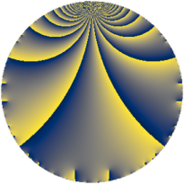Properties

 Label 2394.2.a.tLevel $2394$ Weight $2$ Character orbit 2394.a Self dual yes Analytic conductor $19.116$ Analytic rank $0$ Dimension $2$ CM no Inner twists $1$

Related objects

Newspace parameters

 Level: $$N$$ $$=$$ $$2394 = 2 \cdot 3^{2} \cdot 7 \cdot 19$$ Weight: $$k$$ $$=$$ $$2$$ Character orbit: $$[\chi]$$ $$=$$ 2394.a (trivial)

Newform invariants

 Self dual: yes Analytic conductor: $$19.1161862439$$ Analytic rank: $$0$$ Dimension: $$2$$ Coefficient field: $$\Q(\sqrt{2})$$ Defining polynomial: $$x^{2} - 2$$ Coefficient ring: $$\Z[a_1, \ldots, a_{11}]$$ Coefficient ring index: $$2$$ Twist minimal: yes Fricke sign: $$-1$$ Sato-Tate group: $\mathrm{SU}(2)$

$q$-expansion

Coefficients of the $$q$$-expansion are expressed in terms of $$\beta = 2\sqrt{2}$$. We also show the integral $$q$$-expansion of the trace form.

 $$f(q)$$ $$=$$ $$q - q^{2} + q^{4} + 2 q^{5} + q^{7} - q^{8} +O(q^{10})$$ $$q - q^{2} + q^{4} + 2 q^{5} + q^{7} - q^{8} -2 q^{10} + ( 2 + \beta ) q^{11} + 2 \beta q^{13} - q^{14} + q^{16} + ( 4 + \beta ) q^{17} - q^{19} + 2 q^{20} + ( -2 - \beta ) q^{22} + 4 q^{23} - q^{25} -2 \beta q^{26} + q^{28} + 2 q^{29} + ( -2 - \beta ) q^{31} - q^{32} + ( -4 - \beta ) q^{34} + 2 q^{35} -3 \beta q^{37} + q^{38} -2 q^{40} + ( 2 - 2 \beta ) q^{41} + ( 4 + 2 \beta ) q^{43} + ( 2 + \beta ) q^{44} -4 q^{46} + ( 4 - 3 \beta ) q^{47} + q^{49} + q^{50} + 2 \beta q^{52} + ( -2 - 2 \beta ) q^{53} + ( 4 + 2 \beta ) q^{55} - q^{56} -2 q^{58} + 8 q^{59} + ( -2 - 4 \beta ) q^{61} + ( 2 + \beta ) q^{62} + q^{64} + 4 \beta q^{65} + ( -4 - \beta ) q^{67} + ( 4 + \beta ) q^{68} -2 q^{70} -2 \beta q^{71} + ( -2 - 2 \beta ) q^{73} + 3 \beta q^{74} - q^{76} + ( 2 + \beta ) q^{77} + ( 2 + 2 \beta ) q^{79} + 2 q^{80} + ( -2 + 2 \beta ) q^{82} + ( 6 + 2 \beta ) q^{83} + ( 8 + 2 \beta ) q^{85} + ( -4 - 2 \beta ) q^{86} + ( -2 - \beta ) q^{88} + ( 2 + 4 \beta ) q^{89} + 2 \beta q^{91} + 4 q^{92} + ( -4 + 3 \beta ) q^{94} -2 q^{95} + ( -10 - 3 \beta ) q^{97} - q^{98} +O(q^{100})$$ $$\operatorname{Tr}(f)(q)$$ $$=$$ $$2 q - 2 q^{2} + 2 q^{4} + 4 q^{5} + 2 q^{7} - 2 q^{8} + O(q^{10})$$ $$2 q - 2 q^{2} + 2 q^{4} + 4 q^{5} + 2 q^{7} - 2 q^{8} - 4 q^{10} + 4 q^{11} - 2 q^{14} + 2 q^{16} + 8 q^{17} - 2 q^{19} + 4 q^{20} - 4 q^{22} + 8 q^{23} - 2 q^{25} + 2 q^{28} + 4 q^{29} - 4 q^{31} - 2 q^{32} - 8 q^{34} + 4 q^{35} + 2 q^{38} - 4 q^{40} + 4 q^{41} + 8 q^{43} + 4 q^{44} - 8 q^{46} + 8 q^{47} + 2 q^{49} + 2 q^{50} - 4 q^{53} + 8 q^{55} - 2 q^{56} - 4 q^{58} + 16 q^{59} - 4 q^{61} + 4 q^{62} + 2 q^{64} - 8 q^{67} + 8 q^{68} - 4 q^{70} - 4 q^{73} - 2 q^{76} + 4 q^{77} + 4 q^{79} + 4 q^{80} - 4 q^{82} + 12 q^{83} + 16 q^{85} - 8 q^{86} - 4 q^{88} + 4 q^{89} + 8 q^{92} - 8 q^{94} - 4 q^{95} - 20 q^{97} - 2 q^{98} + O(q^{100})$$

Embeddings

For each embedding $$\iota_m$$ of the coefficient field, the values $$\iota_m(a_n)$$ are shown below.

For more information on an embedded modular form you can click on its label.

Label $$\iota_m(\nu)$$ $$a_{2}$$ $$a_{3}$$ $$a_{4}$$ $$a_{5}$$ $$a_{6}$$ $$a_{7}$$ $$a_{8}$$ $$a_{9}$$ $$a_{10}$$
1.1
 −1.41421 1.41421
−1.00000 0 1.00000 2.00000 0 1.00000 −1.00000 0 −2.00000
1.2 −1.00000 0 1.00000 2.00000 0 1.00000 −1.00000 0 −2.00000
 $$n$$: e.g. 2-40 or 990-1000 Significant digits: Format: Complex embeddings Normalized embeddings Satake parameters Satake angles

Atkin-Lehner signs

$$p$$ Sign
$$2$$ $$1$$
$$3$$ $$1$$
$$7$$ $$-1$$
$$19$$ $$1$$

Inner twists

This newform does not admit any (nontrivial) inner twists.

Twists

By twisting character orbit
Char Parity Ord Mult Type Twist Min Dim
1.a even 1 1 trivial 2394.2.a.t 2
3.b odd 2 1 2394.2.a.u yes 2

By twisted newform orbit
Twist Min Dim Char Parity Ord Mult Type
2394.2.a.t 2 1.a even 1 1 trivial
2394.2.a.u yes 2 3.b odd 2 1

Hecke kernels

This newform subspace can be constructed as the intersection of the kernels of the following linear operators acting on $$S_{2}^{\mathrm{new}}(\Gamma_0(2394))$$:

 $$T_{5} - 2$$ $$T_{11}^{2} - 4 T_{11} - 4$$ $$T_{13}^{2} - 32$$ $$T_{17}^{2} - 8 T_{17} + 8$$

Hecke characteristic polynomials

$p$ $F_p(T)$
$2$ $$( 1 + T )^{2}$$
$3$ $$T^{2}$$
$5$ $$( -2 + T )^{2}$$
$7$ $$( -1 + T )^{2}$$
$11$ $$-4 - 4 T + T^{2}$$
$13$ $$-32 + T^{2}$$
$17$ $$8 - 8 T + T^{2}$$
$19$ $$( 1 + T )^{2}$$
$23$ $$( -4 + T )^{2}$$
$29$ $$( -2 + T )^{2}$$
$31$ $$-4 + 4 T + T^{2}$$
$37$ $$-72 + T^{2}$$
$41$ $$-28 - 4 T + T^{2}$$
$43$ $$-16 - 8 T + T^{2}$$
$47$ $$-56 - 8 T + T^{2}$$
$53$ $$-28 + 4 T + T^{2}$$
$59$ $$( -8 + T )^{2}$$
$61$ $$-124 + 4 T + T^{2}$$
$67$ $$8 + 8 T + T^{2}$$
$71$ $$-32 + T^{2}$$
$73$ $$-28 + 4 T + T^{2}$$
$79$ $$-28 - 4 T + T^{2}$$
$83$ $$4 - 12 T + T^{2}$$
$89$ $$-124 - 4 T + T^{2}$$
$97$ $$28 + 20 T + T^{2}$$